# Separate Strings

Below we will look at a program in Excel VBA that separates strings.
Situation: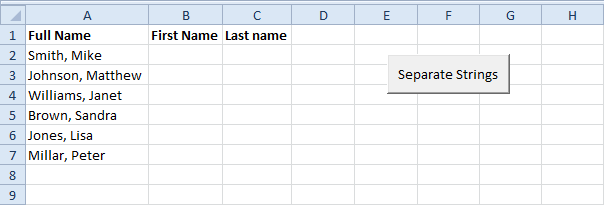Place a command button on your worksheet and add the following code lines:
1. First, we declare a variable called fullname of type String, a variable called commaposition of type Integer, and a variable called i of type Integer.
Dim fullname As String, commaposition As Integer, i As Integer
The problem we are dealing with is that we need to tell Excel VBA where we want to separate the string. In case of Smith, Mike the comma is at position 6 while in case of Williams, Janet the comma is at position 9.
2. We use a loop to execute the operations on each name entered in Excel. First, we initialize the variable fullname. Next, we use the Instr function to find the position of the comma.
For i = 2 To 7
fullname = Cells(i, 1).Value
commaposition = InStr(fullname, ",")
3. Finally, we want to write the part after the comma to Column B and the part in front of the comma to column C. You can achieve this by adding the lines:
Cells(i, 2).Value = Mid(fullname, commaposition + 2)
Cells(i, 3).Value = Left(fullname, commaposition - 1)
Mid(fullname, commaposition + 2) means we want the part of fullname starting at character 'commaposition + 2' (this is exactly the first name).
Left(fullname, commaposition - 1) means we want the part of fullname starting at the beginning until character 'commaposition- 1' (this is exactly the last name).
4. Don't forget to close the loop.
Next i
5. Add six names separated by a comma and space to Range("A2:A7").
6. Test the program.
Result: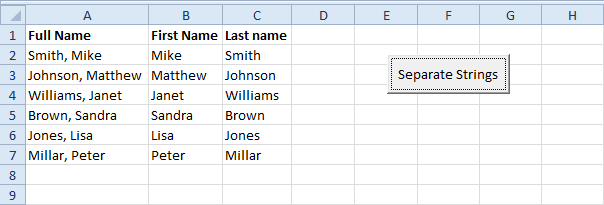### InputBox function in Excel VBA Code

InputBox Function

You can use the InputBox function in Excel VBA to prompt the user to enter a value. Place a command button on your worksheet and add the following code lines:

1. First, declare the variable myValue of type Variant.
Dim myValue As Variant

Note: we use a variable of type Variant here because a Variant variable can hold any type of value. This way the user can enter text, numbers, etc.

2. Add the following code line to show the input box.
myValue = InputBox("Give me some input")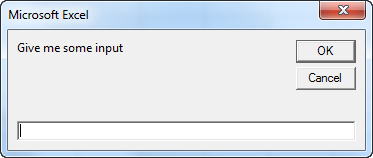3. Write the value of myValue to cell A1.
Range("A1").Value = myValue

Result when the user enters the value 5 and clicks the OK button.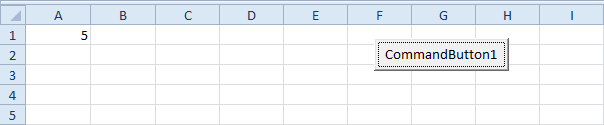4. The InputBox function has more optional arguments. The following code line shows an input box with a title displayed in the title bar and has a default value. The default value will be used if no other input is provided.
myValue = InputBox("Give me some input", "Hi", 1)

Result when the user only clicks the OK button.Note: Place your cursor on InputBox in the Visual Basic Editor and click F1 for help on the other optional arguments.

### VBA Code for excel to send multiple emails with attachments using Outlook

VBA Code for excel to send multiple emails with attachments using Outlook:

Where list of email address in workbook under column "A" and use this VBA code to send an email to each individual email address using MS outlook with the subject in cell "B1", the body message in cell "B2" and for the attachment, full file path in cell “B3”.

This will send an E-Mail to every address in Column "A" starting at row 2 down to the last row.
At the moment it is set to display before sending.
If you don't want to see each E-Mail first, disable the display line and uncomment the Send line.

Sub SendEm()
Dim i As Integer, Mail_Object, Email_Subject, o As Variant, lr As Long, file As Variant
lr = Cells(Rows.Count, "A").End(xlUp).Row
file = Range("B3").Value
Set Mail_Object = CreateObject("Outlook.Application")
For i = 2 To lr
With Mail_Object.CreateItem(o)
.Subject = Range("B1").Value
.To = Range("A" & i).Value
.Body = Range("B2").Value
.Attachments.Add file
'.Send
.display 'disable display and enable send to send automatically
End With
Next i
MsgBox "E-mail successfully sent", 64
Application.DisplayAlerts = False
Set Mail_Object = Nothing
End Sub

### VBA Code for excel to send multiple emails using Outlook

VBA Code for excel to send multiple emails using Outlook:

Where list of email address in workbook under column "A" and use this VBA code to send an email to each individual email address using MS outlook with the subject in cell "B1" and the body message in cell "B2".

This will send an E-Mail to every address in Column "A" starting at row 2 down to the last row.
At the moment it is set to display before sending.
If you don't want to see each E-Mail first, disable the display line and uncomment the Send line.

Code:

Sub SendEm()
Dim i As Integer, Mail_Object, Email_Subject, o As Variant, lr As Long
lr = Cells(Rows.Count, "A").End(xlUp).Row
Set Mail_Object = CreateObject("Outlook.Application")
For i = 2 To lr
With Mail_Object.CreateItem(o)
.Subject = Range("B1").Value
.To = Range("A" & i).Value
.Body = Range("B2").Value
'.Send
.display 'disable display and enable send to send automatically
End With
Next i
MsgBox "E-mail successfully sent", 64
Application.DisplayAlerts = False
Set Mail_Object = Nothing
End Sub

Source

# MsgBox

The MsgBox is a dialog box in Excel VBA you can use to inform the users of your program. Place a command button on your worksheet and add the following code lines:
1. A simple message.
MsgBox "This is fun"
Result when you click the command button on the sheet: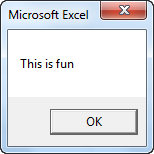2. A little more advanced message. First, enter a number into cell A1.
MsgBox "Entered value is " & Range("A1").Value
Result when you click the command button on the sheet: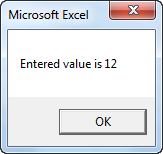Note: we used the & operator to concatenate (join) two strings. Although Range("A1").value is not a string, it works here.
3. To start a new line in a message, use vbNewLine.
MsgBox "Line 1" & vbNewLine & "Line 2"
Result when you click the command button on the sheet: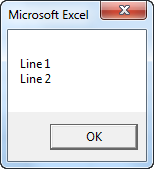## Sunday, February 8, 2015

### VBA Code to Remove Specific Value from a table in MS Excel

Use the Below VBA Code to Remove Specific Value from a table in MS Excel from your active worksheet

`````` Sub RemoveSpecificValue()
'Removing Specific Values whose begins with "FR0"
ActiveSheet.Range("A:G").AutoFilter Field:=1, Criteria1:="=FR0*" _
, Operator:=xlAnd
' Delete AutoFiltered rows except the header
lr = Cells(Rows.Count, 1).End(xlUp).Row
If lr > 1 Then
Range("A2:A" & lr).SpecialCells(xlCellTypeVisible).EntireRow.Delete
End If
End Sub
``````

### VBA Code to Delete Multiple Rows in MS Excel

Use Below VBA Code to Delete Multiple Rows in MS Excel from your active worksheet

Sub DeleteRows()
'Delete the Row 2 and 4 in active worksheet
Rows("2:2,4:4").Select
Selection.Delete Shift:=xlUp
End Sub

### VBA Code to Delete Multiple Columns in MS Excel

Use Below VBA Code to Delete Multiple Columns in MS Excel from your active worksheet

Sub DeleteColumns()
'Delete the Column B and D in active worksheet
Columns("B:B, D:D").Select
Selection.Delete Shift:=xlToLeft
End Sub

### VBA Code to autofit columns in MS Excel

Use Below VBA Code to autofit the columns in MS Excel as per you requirement

Sub AutoFitColumns()
Dim sht As Worksheet

'AutoFit all the Columns in active worksheet
Cells.Select
Cells.EntireColumn.AutoFit

'AutoFit One Column
ThisWorkbook.Worksheets("Sheet1").Columns("O:O").EntireColumn.AutoFit

'AutoFit Multiple Columns
ThisWorkbook.Worksheets("Sheet1").Range("I:I,L:L").EntireColumn.AutoFit 'Columns I & L
ThisWorkbook.Worksheets("Sheet1").Range("I:L").EntireColumn.AutoFit 'Columns I to L

'AutoFit All Columns on Worksheet
ThisWorkbook.Worksheets("Sheet1").Cells.EntireColumn.AutoFit

'AutoFit Every Worksheet Column in a Workbook
For Each sht In ThisWorkbook.Worksheets
sht.Cells.EntireColumn.AutoFit
Next sht

End Sub